EXACTOPTIONS Statement
EXACTOPTIONS options ;

The EXACTOPTIONS statement specifies options that apply to every EXACT statement in the program. The following options are available:

ABSFCONV=value

specifies the absolute function convergence criterion. Convergence requires a small change in the log-likelihood function in subsequent iterations,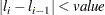whereis the value of the log-likelihood function at iteration.

By default, ABSFCONV=1E–12. You can also specify the FCONV= and XCONV= criteria; optimizations are terminated as soon as one criterion is satisfied.

adds the observed sufficient statistic to the sampled exact distribution if the statistic was not sampled. This option has no effect unless the METHOD=NETWORKMC option is specified and the ESTIMATE option is specified in the EXACT statement. If the observed statistic has not been sampled, then the parameter estimate does not exist; by specifying this option, you can produce (biased) estimates.

BUILDSUBSETS

builds every distribution for sampling. By default, some exact distributions are created by taking a subset of a previously generated exact distribution. When the METHOD=NETWORKMC option is invoked, this subsetting behavior has the effect of using fewer than the desiredsamples; see the N= option for more details. Use the BUILDSUBSETS option to suppress this subsetting.

EPSILON=value

controls how the partial sums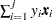are compared. value must be betweenand; by default, value=1E–8.

FCONV=value

specifies the relative function convergence criterion. Convergence requires a small relative change in the log-likelihood function in subsequent iterations,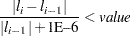whereis the value of the log likelihood at iteration.

By default, FCONV=1E–8. You can also specify the ABSFCONV= and XCONV= criteria; if more than one criterion is specified, then optimizations are terminated as soon as one criterion is satisfied.

MAXTIME=seconds

specifies the maximum clock time (in seconds) that PROC LOGISTIC can use to calculate the exact distributions. If the limit is exceeded, the procedure halts all computations and prints a note to the LOG. The default maximum clock time is seven days.

METHOD=keyword

specifies which exact conditional algorithm to use for every EXACT statement specified. You can specify one of the following keywords:

DIRECT

invokes the multivariate shift algorithm of Hirji, Mehta, and Patel (1987). This method directly builds the exact distribution, but it can require an excessive amount of memory in its intermediate stages. METHOD=DIRECT is invoked by default when you are conditioning out at most the intercept, or when the LINK=GLOGIT option is specified in the MODEL statement.

NETWORK

invokes an algorithm described in Mehta, Patel, and Senchaudhuri (1992). This method builds a network for each parameter that you are conditioning out, combines the networks, then uses the multivariate shift algorithm to create the exact distribution. The NETWORK method can be faster and require less memory than the DIRECT method. The NETWORK method is invoked by default for most analyses.

NETWORKMC

invokes the hybrid network and Monte Carlo algorithm of Mehta, Patel, and Senchaudhuri (1992). This method creates a network, then samples from that network; this method does not reject any of the samples at the cost of using a large amount of memory to create the network. METHOD=NETWORKMC is most useful for producing parameter estimates for problems that are too large for the DIRECT and NETWORK methods to handle and for which asymptotic methods are invalid—for example, for sparse data on a large grid.

N=n

specifies the number of Monte Carlo samples to take when the METHOD=NETWORKMC option is specified. By default, n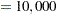. If the procedure cannot obtain n samples due to a lack of memory, then a note is printed in the SAS log (the number of valid samples is also reported in the listing) and the analysis continues.

The number of samples used to produce any particular statistic might be smaller than n. For example, let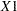and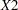be continuous variables, denote their joint distribution by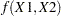, and let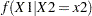denote the marginal distribution ofconditioned on the observed value of. If you request the JOINT test ofand, then n samples are used to generate the estimate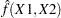of, from which the test is computed. However, the parameter estimate foris computed from the subset ofthat has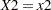, and this subset need not contain n samples. Similarly, the distribution for each level of a classification variable is created by extracting the appropriate subset from the joint distribution for the CLASS variable.

In some cases, the marginal sample size can be too small to admit accurate estimation of a particular statistic; a note is printed in the SAS log when a marginal sample size is less than 100. Increasing n increases the number of samples used in a marginal distribution; however, if you want to control the sample size exactly, you can either specify the BUILDSUBSETS option or do both of the following:

• Remove the JOINT option from the EXACT statement.

• Create dummy variables in a DATA step to represent the levels of a CLASS variable, and specify them as independent variables in the MODEL statement.

NOLOGSCALE

specifies that computations for the exact conditional models be computed by using normal scaling. Log scaling can handle numerically larger problems than normal scaling; however, computations in the log scale are slower than computations in normal scale.

ONDISK

uses disk space instead of random access memory to build the exact conditional distribution. Use this option to handle larger problems at the cost of slower processing.

SEED=seed

specifies the initial seed for the random number generator used to take the Monte Carlo samples when the METHOD=NETWORKMC option is specified. The value of the SEED= option must be an integer. If you do not specify a seed, or if you specify a value less than or equal to zero, then PROC LOGISTIC uses the time of day from the computer’s clock to generate an initial seed.

STATUSN=number

prints a status line in the SAS log after every number of Monte Carlo samples when the METHOD=NETWORKMC option is specified. The number of samples taken and the current exact p-value for testing the significance of the model are displayed. You can use this status line to track the progress of the computation of the exact conditional distributions.

STATUSTIME=seconds

specifies the time interval (in seconds) for printing a status line in the LOG. You can use this status line to track the progress of the computation of the exact conditional distributions. The time interval you specify is approximate; the actual time interval varies. By default, no status reports are produced.

XCONV=value

specifies the relative parameter convergence criterion. Convergence requires a small relative parameter change in subsequent iterations,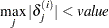where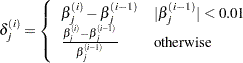and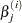is the estimate of theth parameter at iteration.

By default, XCONV=1E–4. You can also specify the ABSFCONV= and FCONV= criteria; if more than one criterion is specified, then optimizations are terminated as soon as one criterion is satisfied.